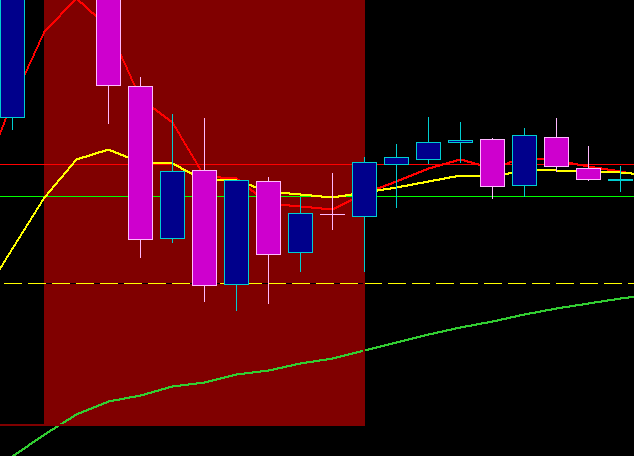# How to make indicator paint behind price

#### TylerDurden

##### New member
Does anyone know how to script the indicator so it will paint behind the price candle? Like the SMA that paints infront of the candle. It would be better if it paints behind the price bar

Last edited by a moderator:
Solution
Following up from almost two years ago (amazing how fast time flies) - this isn't a perfect solution to this old thread, but here's a possible workaround idea.

Code:
``````# Hiding MAs when Price Crosses
# Pensar

input price = close;
input n1 = 10;
input n2 = 20;
input n3 = 50;
input n4 = 100;
input n5 = 150;
input n6 = 200;
input displace = 0;
input averageType = AverageType.SIMPLE;

def n = double.nan;
def h = high;
def l = low;

def mx = max(open,close);
def mn = min(open,close);
plot avg1 = if mx > MovingAverage(averageType, price[-displace], n1) and mn < MovingAverage(averageType, price[-displace], n1) then n else MovingAverage(averageType, price[-displace], n1);
plot avg2 = if mx > MovingAverage(averageType, price[-displace], n2)...``````
@TylerDurden Unfortunately, I dont believe thinkscript can do so. You are able to adjust the transparency of the indicator, though. In the indicator settings, click the color > more > HSV or HSL.

The idea is to have a chart like in the following picture so it's easy to do the price analysis without the MA getting in the way like in the following picture.Last edited by a moderator:
Following up from almost two years ago (amazing how fast time flies) - this isn't a perfect solution to this old thread, but here's a possible workaround idea.

Code:
``````# Hiding MAs when Price Crosses
# Pensar

input price = close;
input n1 = 10;
input n2 = 20;
input n3 = 50;
input n4 = 100;
input n5 = 150;
input n6 = 200;
input displace = 0;
input averageType = AverageType.SIMPLE;

def n = double.nan;
def h = high;
def l = low;

def mx = max(open,close);
def mn = min(open,close);
plot avg1 = if mx > MovingAverage(averageType, price[-displace], n1) and mn < MovingAverage(averageType, price[-displace], n1) then n else MovingAverage(averageType, price[-displace], n1);
plot avg2 = if mx > MovingAverage(averageType, price[-displace], n2) and mn < MovingAverage(averageType, price[-displace], n2) then n else MovingAverage(averageType, price[-displace], n2);
plot avg3 = if mx > MovingAverage(averageType, price[-displace], n3) and mn < MovingAverage(averageType, price[-displace], n3) then n else MovingAverage(averageType, price[-displace], n3);
plot avg4 = if mx > MovingAverage(averageType, price[-displace], n4) and mn < MovingAverage(averageType, price[-displace], n4) then n else MovingAverage(averageType, price[-displace], n4);
plot avg5 = if mx > MovingAverage(averageType, price[-displace], n5) and mn < MovingAverage(averageType, price[-displace], n5) then n else MovingAverage(averageType, price[-displace], n5);
plot avg6 = if mx > MovingAverage(averageType, price[-displace], n6) and mn < MovingAverage(averageType, price[-displace], n6) then n else MovingAverage(averageType, price[-displace], n6);

avg1.SetDefaultColor(createcolor(51,51,51));
avg2.SetDefaultColor(createcolor(51,51,51));
avg3.SetDefaultColor(createcolor(51,51,51));
avg4.SetDefaultColor(createcolor(51,51,51));
avg5.SetDefaultColor(createcolor(51,51,51));
avg6.SetDefaultColor(createcolor(51,51,51));

# end code``````

Last edited:
Last price indicator triggered Questions 1
Golden Zone Indicator Questions 1
Anyone has code for this clouds indicator ? Questions 0
help with pre-market high/low indicator plotting properly Questions 0
Labels Indicator Questions 1

### Not the exact question you're looking for?

87k+ Posts
324 Online## The Market Trading Game Changer

Join 2,500+ subscribers inside the useThinkScript VIP Membership Club
• Exclusive indicators
• Proven strategies & setups
• Private Discord community
• Exclusive members-only content
• 1 full year of unlimited support

What is useThinkScript?

useThinkScript is the #1 community of stock market investors using indicators and other tools to power their trading strategies. Traders of all skill levels use our forums to learn about scripting and indicators, help each other, and discover new ways to gain an edge in the markets.

How do I get started?

We get it. Our forum can be intimidating, if not overwhelming. With thousands of topics, tens of thousands of posts, our community has created an incredibly deep knowledge base for stock traders. No one can ever exhaust every resource provided on our site.

If you are new, or just looking for guidance, here are some helpful links to get you started.

What are the benefits of VIP Membership?